Editor Ratings:
User Ratings:
[Total: 0 Average: 0]

Here are 5 free online trip fuel cost calculator. These websites can help you easily find out the total fuel cost for your trip. To do that, they will ask you to simply enter some trip details like the distance, fuel cost, and the fuel efficiency of the vehicle you’re traveling with. When you’re done with entering these details, the websites will take them into consideration and calculate the fuel cost for you automatically. The best part about these fuel cost calculators is that you can use them for any trip you want regardless of the country you’re traveling in or traveling to.

Many people (like me) use to plan their trip or vacation based on the budget. That means including the fuel cost to the budget is also a critical step, so that you don’t run out of money for the fuel. So, these websites provide you with a simple way to get an estimation of the fuel cost you would require to complete the trip.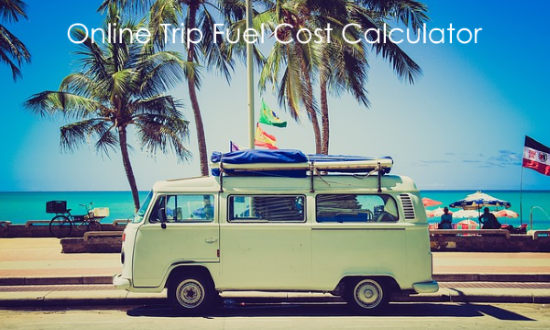## Fuel Cost Calculator (by Roadtrip America):Fuel Cost Calculator (by Roadtrip America) is one of the best free online fuel cost calculator. You can use it to easily calculate the total fuel cost required for your trip. To do that, simply open the website and then you can see the fuel cost calculator. Now, you would need to enter your trip length (either in miles or kilometers), vehicle fuel usage (in miles per gallon or liters per 100 KM), and the fuel price (per gallon or per liter). As soon as you do that, click on the “Calculate” button and then it will automatically calculate the trip fuel cost for you. Additionally, it will also show how much is your fuel cost per mile/kilometer.

## Fuel Cost Calculator (by Calculator.net):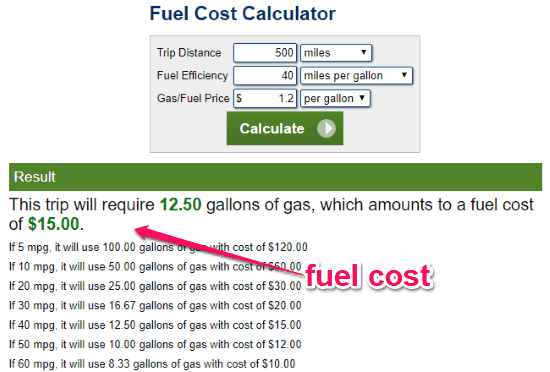Fuel Cost Calculator (by Calculator.net) is another free online trip fuel cost calculator that allows you to calculate the fuel cost required for your trip. It can automatically calculate the cost based on distance, fuel efficiency, and fuel cost. After opening this website, you can see a simple calculator in which you can add these trip parameters. You can enter the trip distance (either in miles or kilometers), fuel efficiency (in miles per gallon, liters per 100 KM, liters per mile, or kilometers per liter), and the gas/fuel price (per liter or per gallon). After that, hit the “Calculate” button and it will instantly show you the trip fuel cost. It will also show you the fuel cost when the fuel efficiency is 5mpg or kmpl, 10mpg or kmpl, 20mpg or kmpl, etc.

## Fuel Cost Calculator (by Everyday Calculation):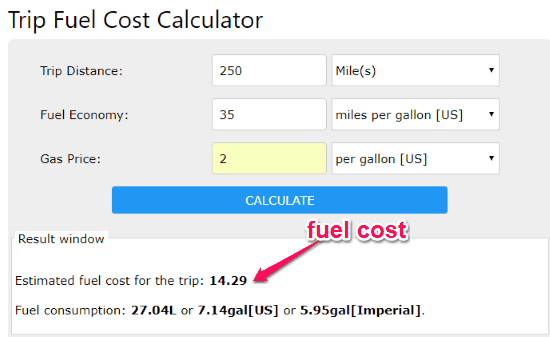Fuel Cost Calculator (by Everyday Calculation) is also a simple online fuel cost calculator for trips. It can automatically calculate the total cost of the fuel you would burn during your trip. For that, you would need to simply open the website and enter some trip-related details. It will ask you to enter the distance of the trip (in kilometers or miles), fuel economy (in miles per gallon US/Imperial, kilometers per liter, liters per 100 kilometers, or gallons per 100 miles), and the gas price (per gallon US/Imperial or per liter). Once you do that, press the “Calculate” button and then it will calculate and show you the total fuel cost instantly. It will also show you how much fuel the trip would consume.

## Fuel & Trip Calculator: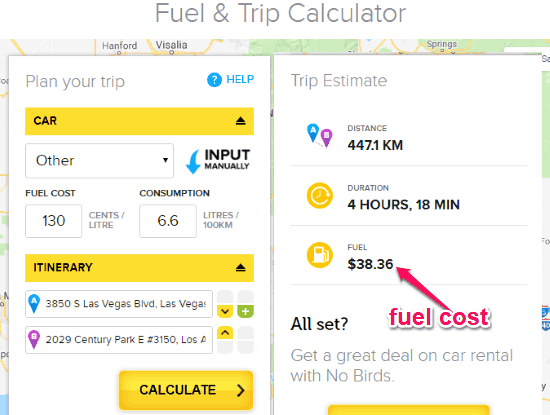Fuel & Trip Calculator can also be used to calculate the trip fuel cost online for free. It also comes with a simple cost calculator in which you would need to enter some details about your trip to calculate the fuel cost. Once you visit this website, you can see the cost calculator on the left. Now, you would need to choose the vehicle you’re traveling with from some pre-defined cars or choose the “Others” option. After that, enter the fuel cost (in cents per liter) and the average fuel consumption (in liters per 100 KM). Then you can move to the “Itinerary” sections and choose the trip’s starting location and the destination. Then click on the “Calculate” button and it will show you the result of the total fuel cost, trip distance you will cover, and the trip duration.

## Fuel Cost Calculator (by Calkoo):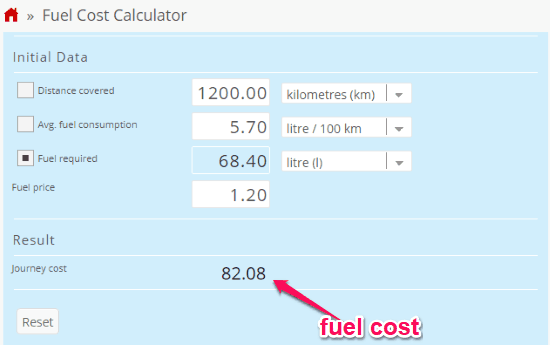Fuel Cost calculator (by Calkoo) is another simple and free online trip fuel cost calculator. It lets you easily find out how much you need to spend on the fuel required for the trip. To begin, simply open this fuel cost calculator and enter the trip’s details under the “Initial Data” section. It will ask you to enter the trip’s distance (in kilometers or miles), average fuel consumption of your vehicle (miles per gallon, miles per liter, etc.), and the fuel price (per gallon or liter). As soon as you’re done with entering these details, it will automatically calculate the fuel cost for your trip and show it to you.

## Closing Words:

So, if you have been looking for a way to calculate the total fuel cost for your trip, then start using any of these free online trip fuel cost calculator. They make it quite easy for anyone to find out about the budget they need for fuel. The results generated by these websites are actually estimations based on the trip details entered by you.